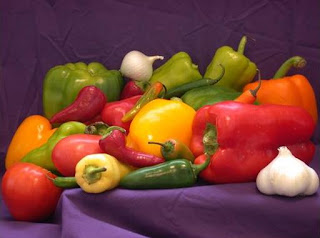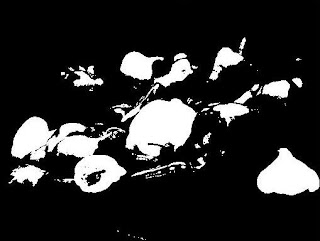## What is Digital image ?

1. Pixel is the smallest element of the image.
2. Pixel is also called as picture element.
3. Digital image is defined as the collection of these picture elements.
4. An image is defined as a two-dimensional function,F(x,y), where x and y are spatial coordinates, and the amplitude of F at any pair of coordinates (x,y) is called the intensity of that image at that point. When x,y, and amplitude values of F are finite, we call it a digital image.

### Types of digital image

• True color image: In true color image, each pixel is composed of 3 components R, G and B i.e. Red, Green and Blue. The dynamic range of intensity value of each component ranges from 0 to 255. The combination of R, G and B with respect to their intensity values creates a new color. Here each pixel is stored as an 24 bit integer (8 bit separately for R, G and B). The Matlab provides a variety of color image dataset in its database, that can be shown using below code.
figure;
imshow(XRGB)Fig 1. True color image
• Gray scale image:  In gray scale images, the dynamic range of intensity value of each pixel ranges from 0 to 255. Here, the pixel is stored as 8 bit integer. The Matlab provides an unique in-built function "rgb2gray" that converts any color image to grayscale image.
figure;
imshow(XRGB)
gr = rgb2gray(XRGB)
figure;
imshow(gr)Fig 2. Gray scale image
• Binary image: In binary images, the dynamic range of intensity value of each pixel ranges from 0 to 1. Here, the pixel is stored as 1 bit integer. The Matlab provides an unique in-built function "im2bw" that converts any intensity (color or grayscale) image in to binary image.These images are also called as "monochrome" and "black and white" images.

figure;
imshow(XRGB)
gr = rgb2gray(XRGB)
figure;
imshow(gr)
bw = im2bw(gr);
figure;
imshow(bw)Fig 3. Binary image
Color Image Processing: Why ? How ?
1. In most of the research papers it is observed that the major portion of research in image processing is performed on the gray scale image.
2. It is frequently observed that these researcher converts the color image dateset into gray scale and then process them.
3. The main  reason of this conversion is easy to understand. The processing of gray scale images are easier than processing of color images due to single channel. In gray scale images, the researcher have to process pixel of 8 bit while in color images, the researchers have to process pixel of 24 bit (8 bit each for Red, Green and Blue). Therefore processing three different channels i.e. R,G and B is comparatively difficult than single channeled gray scale images.
4. It is important to not change the real nature of original color image because after converting any color image into gray scale image, although the processing becomes easy but a very useful and necessary information may lost.
5. Sometimes this loss of information results in to a failed application.
6. The processing of color image is same as processing as gray scale images, only the need is to subdivide the color image into three components R, G and B and then process them like  a gray scale image. Lastly after processing the modified R, G and B, they are fused backed to regenerate original color image. Below code explains the processing of color images:
figure;
imshow(i)

// i is the read color image. The below code divides the color image i into three different channels i.e. Red, Green and Blue channels. Now each channel (Red, Green and Blue) can be processed in same way the gray scale image is processed.
modR = i(:,:,1);
modG = i(:,:,2);
modB = i(:,:,3);

// Now process each channel (Red, Green and Blue) in same way the gray scale image is processed. Because now each channel (Red, Green and Blue) becomes like a gray scale image as their intensity range varies from 0-255.
processedR = processImage(modR);
processedG = processImage(modG);
processedB = processImage(modB);

// The processedR, processedG, and processedB are the processed three channels. Now an in-built function i.e. "cat" is used to concatenates the arrays processedR, processedG, and processedB along dimension 3. This is a inverse process to get back processed color image.
finalRGB = cat(3,processedR, processedG, processedB);

// finalRGB is the final processed color image.
figure;
imshow(finalRGB)

For more image processing topics refer: https://www.gofastresearch.com/

What is Digital image and Color Image Processing in MATLABReviewed by IPR on April 24, 2020 Rating: 5